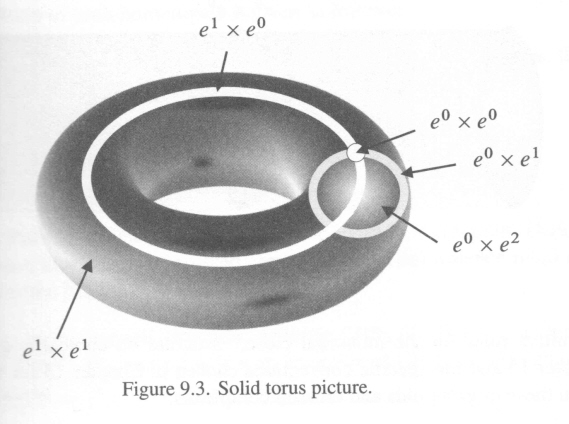# Complement of the Solid Torus in $S^3$ is Again a Solid Torus

On pg. 48 of Hatcher's Algebraic Topology, the author writes that the $3$-sphere $S^3$ can be thought of as the union of two solid torus.

First a formal reasoning is given which is

$S^3=\partial D^4 = \partial(D^2\times D^2) = (\partial D^2\times D^2)\cup (D^2\times \partial D^2)$

This is clear. But then the author gives a geometric interpretation which is as follows.

Think of $S^3$ as the one point compactification of $\mathbf R^3$. Let $T=S^1\times D^2$ be the first solid torus. The second solid torus is the closure of the complement of $T$ in $\mathbf R^3$ along with the compatification point at infinity.

The statement in italics is not clear to me. How do we see this as a solid torus?

• One nice way to see the symmetry is to rotate $S^{3}$ so that the north pole lies on the boundary torus; stereographic projection sends the torus to a "parabolic ring cyclide" (q.v.), and (for a Clifford torus) the two "sides" are congruent subsets of $\mathbf{R}^{3}$. That Wikipedia page has a loop animation by Jason Hise, which should help visualize matters. Jan 21, 2016 at 15:44
• I am not able to follow your idea but I would certainly like to learn this approach. Can you frame this as an answer? Jan 21, 2016 at 23:18

Here's another way that I like to view this: The solid torus $S^1 \times D^2$ is a donut, and you can fill in the donut hole with a plug ("munchkin" if you're a Dunkin' Donuts fan) homeomorphic to $D^2 \times [-1,1]$. The union of these is isotopic to the standard $D^3 \subset \mathbb{R}^3 \subset S^3$, so the complement (of its interior, technically) is also homeomorphic to $D^3$. The plug $D^2 \times [-1,1]$ intersects this outer $D^3$ along $D^2 \times \{\pm 1\}$. Working in reverse, we see that the complement of the original solid torus is obtained by attaching $D^2 \times [-1,1]$ to the outer $D^3$, together forming a complementary solid torus.

• By 'isotopic' do you mean 'homemorphic'. Further, the complement of $D^3$ in $S^3$ doesn't seem to be exactly $D^3$. Probably you mean that the closure of the complement of $D^3$ in $S^3$ is $D^3$. Or perhaps I misunderstand you. Thanks. Jan 21, 2016 at 23:07
• @caffeinemachine: I do mean `isotopy' here, which is a stronger notion. If I have a subspace of $S^3$ that is only homeomorphic to $D^3$, then the closure of its complement will not necessarily be homeomorphic to $D^3$. Take for example, the ball bounded by the Alexander horned sphere. However, the closure of the complement of any "locally-flat" embedding of $D^3$ in $S^3$ will indeed be homeomorphic to $D^3$, and that is the case here.
– Kyle
Jan 21, 2016 at 23:23
• This makes things clear. I was thinking on the same question. Whether it is necessary that the closure of the complement of $D^n$ in $S^n$ is again a $D^n$. But you gave me Alexander horned space. Jan 21, 2016 at 23:25

The following picture (from this book) may help:Now imagine a vertical line through the centre of the "hole" and a whole family of circles around the torus, expanded and rotated versions of the $e^0 \times e^1$. The vertical line with a point at infinity is also a circle.

Another way of seeing this, equivalent to the answer of Kyle, is to say that the 3-sphere is given by the subset of $\mathbb R^4$ given by $x{_1}^2 + x_2{^2} + x_3{^2} + x{_4}^2=1$. One of the solid tori is given by $x{_1}^2 + x_2{^2} \leqslant 1$.

• I am still not able to see why would this give us a complementary torus. Could you please try to say something more about this. Thank you. Jan 21, 2016 at 23:16
• I tried to find a movie of this, but I did find dimensions-math.org/Dim_CH7_E.htm on the Hopf map which could help your imagination. Try drawing a picture of the $xz$-plane, cutting the solid torus in 2 disks. The $z$-axis is a vertical line. Then on each side of this we have a family of circles round the two disks, getting larger and larger, and "nearer" to the $z$-axis.. This picture then rotates about the $z$-axis. Jan 22, 2016 at 17:10
• Jan 22, 2016 at 17:12